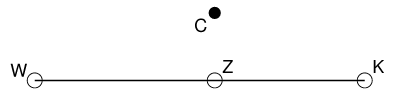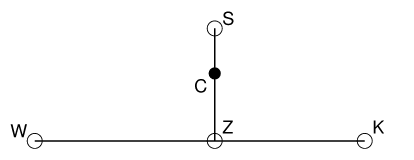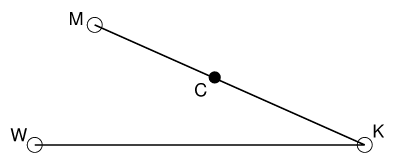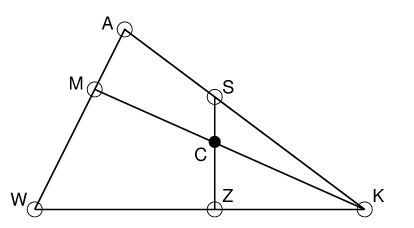# Colour Contrast Enhancement

This document describes the method used by the Adaptive Contrast Enhancement (ACE) package for colour images. (Note that this is only implemented for the command-line and Tk versions of the software; the GIMP plug in may be updated once a decision is made. The pictures in my web pages were generated using this method.)

The basic form of most colour contrast enhancement starts with the conversion of the image into grayscale, such as by taking the luminance values. Grayscale enhancement is applied to this image. Then the new and old grayscale values at each point are used in a transformation of the pixel colour. The design decisions are how to convert to grayscale and then how to use the enhanced gray value to transform a colour. There are widely differing choices.

We are concerned with aesthetic image enhancement. Therefore, if we enhance an image which happens to be completely blue, we expect the output to be blue as well. If the colour image were completely gray, we would expect ACE to leave it that way, and preferrably to transform it in exactly the same manner as grayscale ACE would.

Therefore, we expect colour transformation to leave hues unchanged, to make modest changes to saturations and to primarily affect the luminance. In the following analysis we work with the idea that, if all input pixel colours were to lie along a certain line in the colour space, then the output pixel colours should do so also. ACE would just slide the colours along this line. We associate exactly one such line with every point in the colour space. In other words, given a colour we can find the line to which it belongs. The only exception is black: all lines start at 100% black.

That sounds complicated, so we should move to specifics. We will use an RGB colour space. Imagine a colour C in colour space. If we converted the image to grayscale by the most common method (taking the luminance component), we would have a zero-saturation colour Z. All these gray colours Z lie on a line between black (K) and white (W):There is a line of colours from Z through C with varying saturation but the same hue and luminance. The saturation is limited, so we can find the colour S with the maximum saturation:We can draw another line through C, this time starting at black and proceeding until we achieve the colour M with maximum luminance:We can generate colours on this line simply by multiplying the r, g and b values by the same factor. The colour M is obtained using the maximum scale factor which generates a valid colour triplet. Therefore, we are limited by the maximum value of the three components r, g and b. Let's put all these lines together:We have completed the figure, making a triangle between B, K and a new colour A. This is the colour with the maximum saturation for the same hue and any luminance.

Colour ACE takes the ratio of the distances K to C and K to M as the input x to the grayscale enhancement. That is, x= KC/KM= max(r,g,b). The ratio of the output y to the input is used to scale the colour. That is, r-> (x/y)*r, and so on.

It would be possible to use this paradigm with other colour systems and gamuts. For a given colour C we could identify the space of colours with the same hue, and this will include the K-B line as an edge. We would then need to define, for each hue, a `maximum saturation' point A and a set of lines (probably curves) all starting at K and ending somewhere along the W-A limit. These lines would range between K-B and K-A.